# VBA Do Loop

A snippet of code that runs a condition repeatedly until a certain criterion is triggered.Reviewed by

Christopher Haynes

Expertise: Asset Management | Investment Banking

Updated:

January 4, 2023

A ‘Do Loop’ is a snippet of code that runs a condition repeatedly until a specific criterion is triggered.Primarily there are two different ‘Do Loops’ in VBA - Do Until and Do While.

The ‘Do Until’ code repeats a condition ‘until’ that condition is met. The easiest example to explain this is counting numbers(do) until we reach the first two-digit numerical value. We know that the first two-digit number is 10.

Thus, the condition will count the numbers from 1,2,3…. to 10 and finally stop since we have fulfilled the expected criteria.

On the other hand, we have the ‘Do While’ subsection that repeats the condition until it is triggered as FALSE. For example, count the numbers(do) while they are still a single digit.

This will count all the numbers from 1,2,3…8 and end the Loop on nine since the subsequent number is a two-digit value.

Both loops are essential in automating manual tasks since each plays a pivotal role in handling the data.

In this article, we will see how both the loops work and how you can use them in your code to make the macro even more dynamic.

## What is a VBA code?

Before we understand how the ‘do loop’ works, it is essential to understand a VBA code. VBA stands for Visual Basics for Application. Does Microsoft develop a programming language for Microsoft Office Applications such as Ms. Excel, Ms. Word, etc.?In simple terms, if you do a repetitive task daily, VBA code will let you automate that piece of work. For example, copying the data from file A to file B with just a click of a mouse.

The program eliminates human intervention, such as opening the workbooks, copying the data, pasting the data, and closing both workbooks.

So far, it does sound fun to work on VBA and automate the workflow. We don’t intend to scare you but building a VBA code can sometimes be tricky.

You need to visualize the flow of how the code should run, break down each component of the task and write the code accordingly.

But it’s alright! We guarantee that with a bit of practice, literally anyone can master this great tool and enjoy longer coffee breaks while you have already completed your work (just don’t let your boss know about it)

## Do While Loop

A ‘Do While’ loop keeps repeating a condition until a criterion is fulfilled or a particular value is obtained.The simplified syntax for the Loop is:

Do While condition

A statement that needs to be executed

Loop

where,

• While condition - the criteria up to which the Loop keeps iterating
• Statement - the snippet of code that gets executed every time the condition is fulfilled.
• Loop - tells the code to run back to the ‘Do while’ Loop to check if the condition is still fulfilled.

## NOTE

You can input multiple conditions between the loop statements, which get fulfilled in the given chronological order.

All three components work together to give what we call a ‘do while’ Loop. Let’s see a simple example of how a ‘do while’ Loop works.

Suppose you need to return all the numbers from 1 to 10 in a single column in Excel. The snippet of code that we will use to get the numbers is: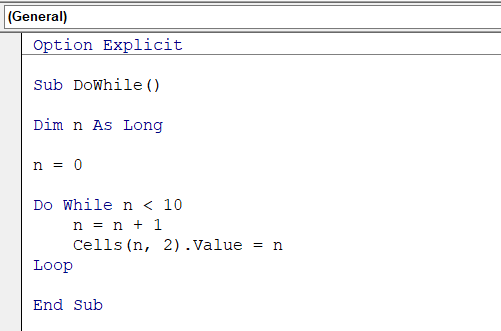This gives us the result in column B asFirstly, we define a variable ‘n’ which will be used as a counter to count the numbers from 1 to 10.

Then, we set up two conditional statements - ‘while n < 10 and n = n + 1’. The first condition means that all the numbers in the Loop will be less than 10, i.e., 0, 1, 2, 3….9.

These numbers will then be used in the following condition to solve the equation. For example, n = 0 + 1 equals 1. Similarly, n = 1 + 1 equals 2, and so on.

Finally, we input the cell reference where we want to input the value, which is a dynamic code Cells(n, 2). Value = n.

When you assign the looping variable ‘n’ as the row_number, the VBA code automatically switches to the next row when the next Loop runs and assigns the respective number.

Once the entire Loop runs, we get the numbers from 1 to 10 in column B.

## NOTE

Different data types can be used to store numeric values in VBA. Long can be used to store larger numbers as opposed to the integer, but the former's drawback is that it takes longer to process.Begin your journey into Excel modeling with our free Excel Modeling Crash Course.

## Do Until Loop

A do-until loop is a looping construct in VBA that allows you to repeat a block of code until a certain condition is met. The do-until Loop is similar to the do-while Loop, but the condition is checked at the beginning of each iteration instead of at the end.

Here is an example of a simple do-until loop in VBA: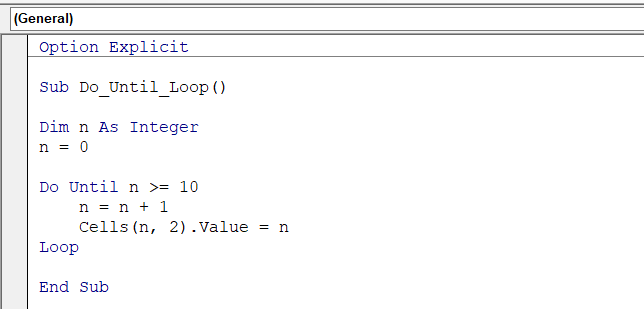This code will start the counter of n from 0 to 9. The Loop continues as long as the condition n >= 10 is false, and the value of n is incremented by 1 at the beginning of each iteration using the statement n = n + 1.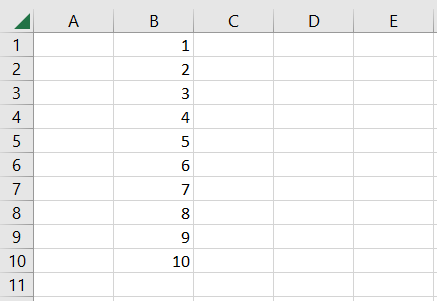The Loop will terminate when the condition becomes true, at which point n will equal 10.

You can also use a do-until loop with the Until keyword followed by an adverse condition, like this: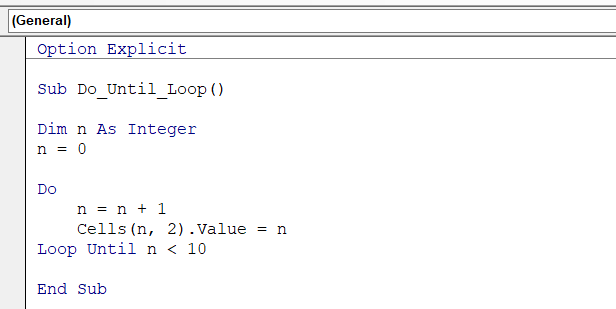This code will have the same behavior as the previous example, but the condition is negated and checked at the end of each iteration.

## NOTE

The snippet of code is essential but won’t work without the Sub…End Sub syntax. If you forget to write either of those lines in the VBA code, your program won’t work.

## Using the Do Loops Together

It is possible to use both do-while and do-until loops in the same VBA code as long as they are appropriately nested within each other or are used in separate blocks of code.

Here is an example of how you can use both do-while and do-until loops in the same code: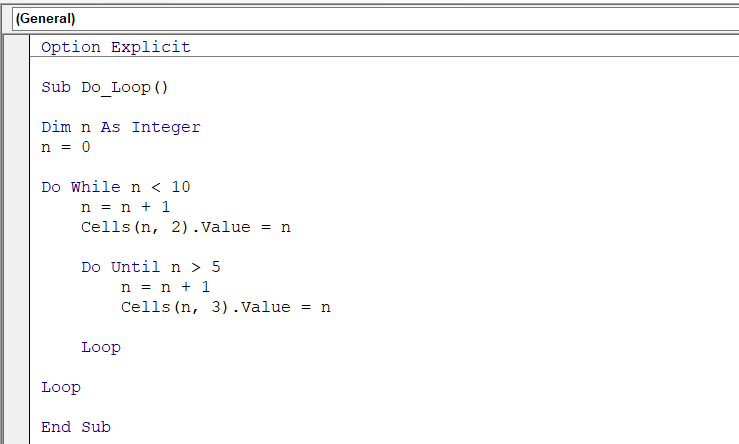In this example, the outer do-while Loop will continue if n is less than 10.

Inside the Loop, a nested do-until loop will continue if n is less than 5. The Inner Loop will increment n by 1 each time it is executed, so it will run 5 times before the condition becomes true and the Loop terminates.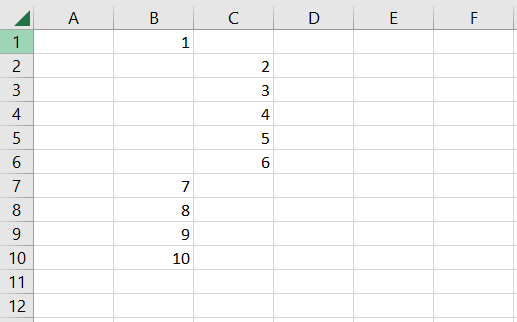After the inner Loop finishes, the control returns to the outer Loop, continuing as long as the condition n < 10 is true.

You can also use do-while and do-until loops in separate blocks of code like this: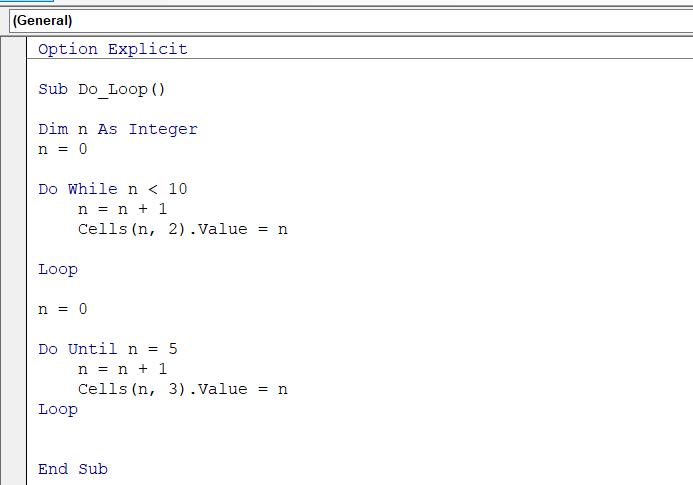In this example, the first do-while Loop will print out the values from 1 to 10, and the second do-until Loop will print out the values from 1 to 5.

For each Loop, the counter value i.e., the value of ‘n,’ begins from zero until the condition of the Loop is met to return the numbers in the spreadsheet as illustrated below: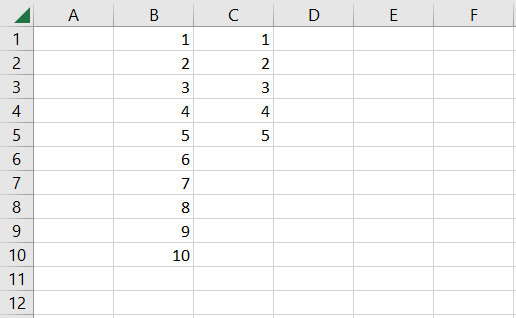## NOTE

If you intend to exit the Do loops early, you can use the ‘Exit Do’ statement at the end of the Loop. This ensures that if a specific condition is met somewhere in the middle, the Loop doesn’t continue until the end and exits early.### Everything You Need To Master Excel Modeling

To Help You Thrive in the Most Prestigious Jobs on Wall Street.

## Do Loops in real-world scenarios.

This section gives an example of how you might use do-while and do-until loops in a real-world scenario in VBA:

Imagine you have a spreadsheet with a list of names in column A and a list of ages in column B. You want to write a macro that will go through the list and print out the names of all people over 18 years old.

The data looks as illustrated below:Here is how you could do it using a do-while loop: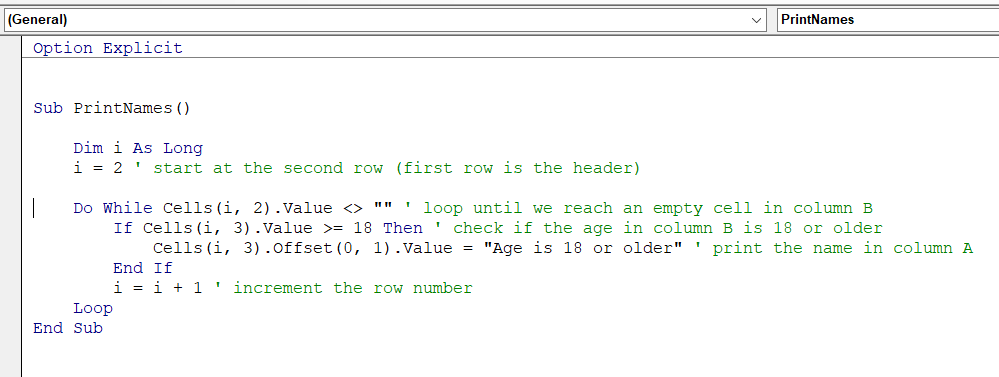When we run the code, the do-while Loop evaluates all the cells in column B to check if it is empty. If a cell is found empty, the Loop immediately ends.

If the Loop isn’t empty, the Loop moves to the following condition to check whether the age in column C is equal to 18 or older.

Whenever the condition evaluates to TRUE, we get a customized text string as a result in column D, or else the cell is left empty if the condition is FALSE, as in the case of cell C3 illustrated below:## Do Loop vs. For Next Loops

A For Loop allows you to repeat a block of code several times. The syntax for a For Loop is:

For i = start To end

'Code to be executed

Next i

Here, the Loop will start with the variable "i" equal to the value of "start" and will continue until "i" reaches the value of "end". The Loop will execute the code inside the Loop once for each value of "i," with "i" increasing by 1 each time the Loop is completed.

Both Make Loops and For Loops can be useful for automating tasks in Excel, such as formatting data, calculating values, and creating reports.

However, Do Loops are better suited for situations where you don't know how many times the Loop needs to be executed, while For Loops are better suited for situations where you need to repeat a task a specific number of times.

Let's see an example of using the For Loop in Excel VBA to format a range of cells. The VBA code that we will use is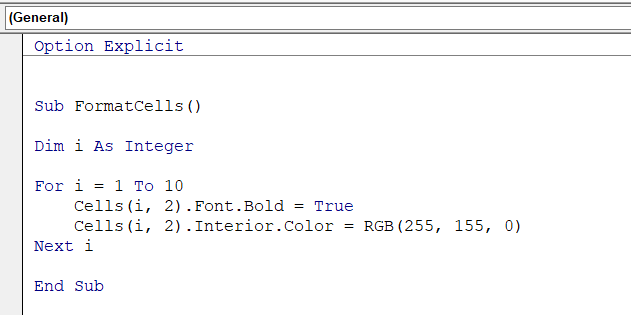This VBA code defines a subroutine called "FormatCells" that uses a For Loop to format the cells in the second column of a worksheet.

The Loop starts with the variable "i" equal to 1 and continues until "i" reaches the value of 10. The code inside the Loop sets each cell's font to bold and the cell's background color to red.

To use this code, you need to open the VBA editor in Excel (by pressing Alt + F11) and write the code into a new module. Then you can run the code by pressing the "Run" button or by pressing F5.

You will find that the code formats the first ten cells in the second column of the active worksheet, as illustrated below.## NOTE

Use a ‘For loop’ when you know that the Loop should be executed ‘n’ several times, whereas the ‘Do While’ loop should be used when you are unaware of how many times the Loop should iterate through data.### Everything You Need To Master Excel Modeling

To Help You Thrive in the Most Prestigious Jobs on Wall Street.

Researched and Authored by Akash Bagul | Linkedin

Reviewed & Edited by Ankit SinhaLinkedIn